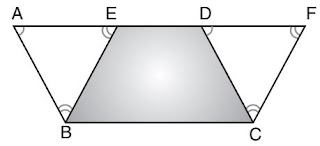## Revision Notes of Chapter 9 Area of Parallelograms and Triangles Class 9th Math

Topics in the Chapter
• Area of Plane Figures
• Fundamentals
• Theorems
Areas of Plane Figures
• Two congruent figures have equal areas.
• A diagonal of a parallelogram divides it into two triangles of equal area.
• Parallelograms on the same base (or equal base) and between the same parallels are equal in area.
• Triangles on the same base (or equal bases) and between the same parallels are equal in area.
• Area of a parallelogram = base × height.
• Area of a triangle = 1/2 ×base × height
• A median of a triangle divides it into two triangles of equal area.
• Diagonals of a parallelogram divides it into four triangles of equal area.
Fundamentals
• Two figures are said to be on the same base and between the same parallels, if they have a common base (side) and the vertices (or the vertex) opposite to the common base of each figure lie on a line parallel to the base.

Examples:• Area of triangle is half the product of its base (or any side) and the corresponding altitude (or height).
• Two triangles with same base (or equal bases) and equal areas will have equal corresponding altitudes.
• A median of a triangle divides it into two triangles of equal areas.

Theorems

Theorem 1:
Statement: Parallelograms on the same base and between the same parallels are equal in area.

Given: Two parallelograms ABCD and EBCF on the same base BC and between the same parallels BC and AF.

To prove: ar (||gm ABCD) = ar (||gm EBCF)

Proof:In ΔABE and ΔDCF,
EAB = ∠FDC (pair of corresponding angles are equal as BE||CF, AF is the transversal) --- (i)
FDC =
∠BEA = ∠CFD (pair of corresponding angles are equal as BE||CF, AF is the transversal) --- (ii)
Now,
∠EAB + ∠ABE + ∠BEA = ∠FDC + ∠DCF + ∠CFD = 180° (sum of measure of the interior angles of a triangle is 180°)

∴ ∠ABE = ∠DCF --- (iii) [using (i) and (ii)]
Also, AB = DC (Opposite side of a ||gm are equal) --- (iv)
Using (i), (iii) and (iv),
ΔABE ⩭ ΔDCF  (ASA rule)
∴ ar (ΔABE) = ar (ΔDCF)
∴ ar (||gm ABCD) = ar (ΔABE) + ar (trapezium EBCD)
= ar (ΔDCF) + ar (trapezium EBCD)
= ar (||gm EBCD)
Hence, ar (Il gm ABCD) = ar (||gm EBCF)

Theorem 2:

Statement: Two triangles on the same base (or equal bases) and between the same parallels are equal in area.

Given: Two triangles ΔABC and ΔDBC are on the same base BC and between the same parallels EF and BC.

To prove: ar(ΔABC) = ar(ΔDBC)

Construction: Through B, draw BE || AC, intersecting the line AD produced in E and through C, draw CF || BD, intersecting the line AD produced in F.Proof :
EACB and DFCB are parallelograms (since two pairs of opposite sides are parallel).
Also ||gm EACB and ||gm DFCB are on the same base BC and between the same parallels EF and BC.
ar (||gm EACB) = ar (||gm DFCB) --- (i)
Now,
AB is the diagonal of ||gm EACB
∴ ar(ΔEAB) = ar(ΔABC)
∴ ar (ΔABC) = (1/2) ar(||gm EACB) --- (ii)
Similarly,
ar (ΔDBC) = (1/2) ar(||gm DFCB) --- (iii)

From equations (i), (ii) and (iii), we get,
ar(ΔABC) = ar(ΔDBC)

Theorem 3:

Statement: Two triangles on the same base (or equal bases) and equal areas lie between the same parallels.